## 1. Introduction

There are many occasions in which generating random numbers is helpful – computer games being a key example, statistical analysis, cryptography, and many others.

The generation of each random number can often be considered a unique event. That is, it has no connection to past or future events. If we imagine rolling a dice, every single roll is a unique event and has an equal chance of being any valid number regardless of all past rolls of the same dice.

In some cases, though, we want to constrain our random numbers. A pervasive constraint ensures that the exact number is never generated more than once. For example, if we simulate a shuffled deck of cards or select songs from a playlist, we want to ensure that each one is chosen exactly once.

In this tutorial, we’re going to learn about a number of techniques to easily allow us to generate random numbers such that each number is only generated once.

## 2. Recording History

The easiest way to achieve this is to record all past events and compare any new number to that set. This way, we can make certain that every number generated has never been seen before:We can clearly see that this will never return the same number twice – every time we ask for a new number, it will keep looping until it randomly selects one that has not been seen before, records it in the history, and only returns it.

However, it will be very inefficient in both time and memory usage. The first number generated will be unique because we’ve never seen any before. However, by the time we’re generating number 100 we’re now storing 99 previous values, we have to check if any of those 99 numbers matches the one we’ve just generated, and we need to keep generating numbers until this new one is unique.

As we reach the limit of the numbers we’re generating, this will get slower and slower. In fact, the algorithm works in O(2n) time. If we’re trying to generate 100 unique numbers in the range 1-100, the very last one will have to be the only one that hasn’t yet been seen, which could take an unknowable amount of time to generate.

## 3. Pre-Shuffling Numbers

The result of our previous algorithm is that the history collection is a fully shuffled list of numbers. Given this, one alternative we have is to reverse the algorithm. We can first generate a shuffled list of numbers, and then generating our random numbers is done by pulling the next number from this list:Immediately we can see that generating each number will be efficient. It’s just pulling the next number from a list, which is an O(1) operation. However, we have an expensive cost up-front of generating the shuffled list. In fact, if we’re generating a comparatively small number of values from a larger set, then this up-front cost will dominate.

## 4. Shuffling During Generation

So far, we’ve seen two algorithms, one with a considerable up-front cost and an efficient way to generate numbers, while the other has no up-front cost but is very expensive to generate numbers. However, we can combine these to have no up-front cost and still be efficient for generating numbers.

We do this by shuffling the numbers as we generate the random numbers, instead of doing it beforehand. This is done by keeping track of how many numbers we’ve generated so far, and for each new number, we pick a random one from the unused set, swap it into the used set and then return it.This looks more complicated than earlier, so let’s step our way through it by generating a couple of random numbers and see how it works.

To start with, we generate a list of numbers and set our count to point to the end of the list: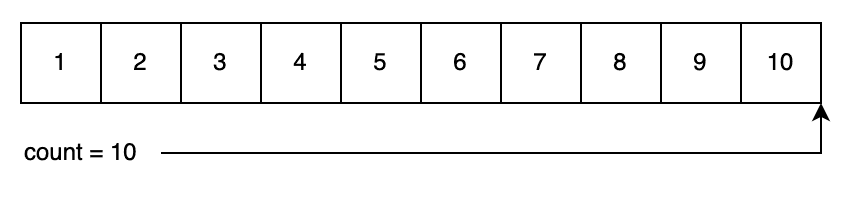Now we’ll generate our first random number. We pick a random index between 0 and our count – 10. In this case, we picked “1”. Now we decrement our count – so that it points 1 short of the end – and then swap our generated index for the index that our counter is pointing to. Then we return the number that our counter is pointing to: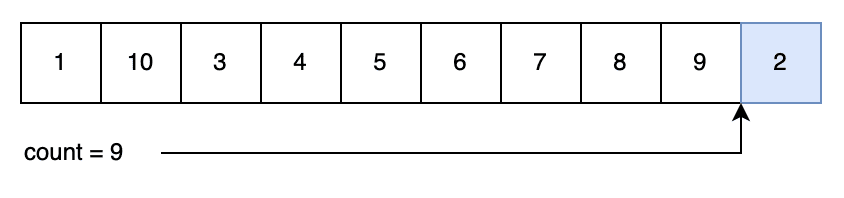So we’ve now generated our first random number – “2” – and we’ve got nine numbers left to pick from.

So let’s do it again. This time we randomly pick “5”, so we’ll decrement our count, swap our values and return them again: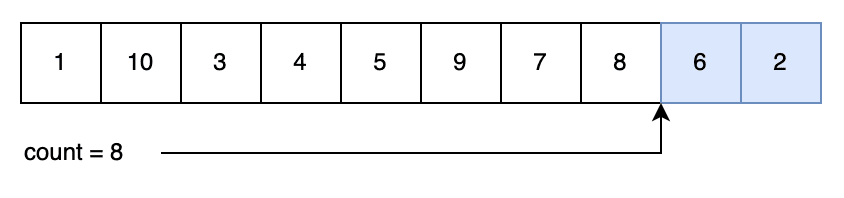It’s important to note that the order in which we generate our index and decrement our count ensures that we can select any of the remaining numbers. For example, imagine our next random index was “7”.

We’d then decrement the count, swap our randomly generated index “7” for our new counter target of “7”, and then return the value “8” that is now in this index to the caller: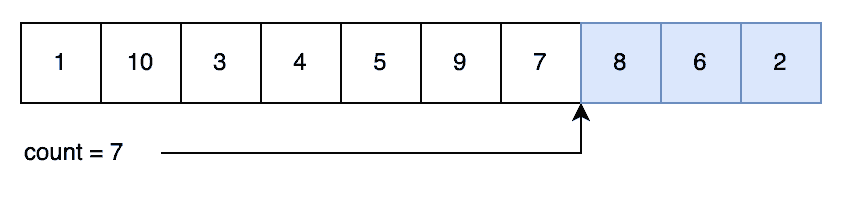Eventually, we’ll get into a situation where we select the same random index as before. But that’s fine because that index now has a different number – that we haven’t yet used – in it, so using that will still generate a unique number.

For example, if we now randomly select index “1” again. This actually contains the number “10” – because it was swapped when it was first used. So this time, we’ll swap the “10” into the correct position – which currently contains “7” – and then return the “10”. So we’ve successfully generated another unique number: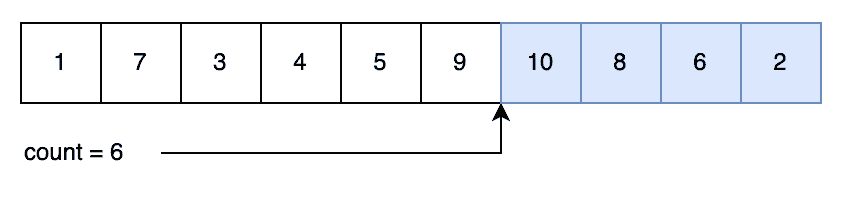So we now have an algorithm that will generate unique random numbers in O(1) time, but without having the high up-front cost of shuffling the numbers first.

## 5. Can We Do Better?

So far, we’ve discussed an algorithm that will produce unique random numbers in O(1) time, which is fantastic. However, it still needs to have significant memory usage. It requires an array large enough for every number we can generate and a counter for how many we’ve generated so far.

In small cases, this memory usage is negligible. If we only want to generate numbers to represent playing cards, for example, then we need memory to store 53 (52 cards + the counter) numbers. Using 32-bit numbers for convenience, which is much larger than is needed, would result in 212 bytes of memory.

However, what if, instead, we want to be able to generate any 32-bit integer? This would mean that we need a single 32-bit counter and an array with one entry for every possible 32-bit integer. This means we need (232+1)*4 bytes of memory, which is ~16GB.

So how do we avoid this? The only option is not to store an array of every possible number. This means that we can’t use the second or third algorithm that we’ve seen here. We could use the first one because that only stores the generated numbers. That means our memory usage is limited by the set of numbers we’ve generated, not the set of numbers we can generate from.

There are some alternative options that could be used – for example, generating cryptographic hashes of a counter. However, these algorithms are only pseudorandom at best, which will probably be good enough for most uses. We also can’t guarantee that the numbers are unique if we don’t have a history of what we’ve generated before.

## 6. Summary

Here we’ve seen a few algorithms that can generate unique random numbers from the desired set, with options that optimize for memory usage, execution time, and code complexity.

In most of these cases, we are assuming that we have a source of true random numbers – for example, /dev/random on Linux has an underlying entropy pool, so is significantly more random than many other choices. However, they will work perfectly well regardless of the quality of the random number source – the only difference is that the quality of the output will be directly related to the quality of the inputs.

So, The next time you need to use random numbers and ensure they are non-repeating, why not consider one of these.